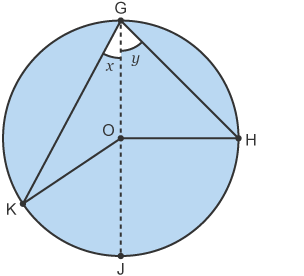墨滴Opsimath

2021/09/08  阅读：31  主题：默认主题

# Mathematics Interview Questions (IX)

### 41. Next you are given a quadrilateral with fixed perimeter and you want maximise the area. What shape will it be? Prove it.

Solution. A square. We will first prove that it is a rectangle, then a square.

Of course, the quadrilateral must be convex. Suppose it is . Then its area is the sum of the areas of and or the sum of the areas of and .

Since the area of a triangle can be expressed as where is between 0 and 1, the area of . Hence, the area of the quadrilateral is and . Adding them together, we get , which, from the AM-GM inequality, .

The first equality is achieved when all angles are right angles, so the quadrilateral is a rectangle; the second equality is when , so it must be a square.

### 42. Integrate .

Solution. The first one: , so , and .

The second one: , so , and .

### 43. How many 0's are at the end of 100! ?

Solution. Each pair of 2 and 5 produce a 0. In 100!, there are much more 2's than 5's, so considering 5's only would be acceptable. Each number with a factor of 5 produce one 5, and 25,50,75,100 produce an extra 5. There are 20+4=24 5's; therefore, there are 24 0's at the end of 100! .

### 44. Prove that the angle at the centre of a circle is twice that at the circumference.

Solution.Let angle OGH = and angle OGK = .

Angle OGH = angle OHG because triangle GOH is isosceles; Similarly, angle OGK = angle OKG because triangle GOK is also isosceles.

Hence, angle JOK = and angle JOH = , which means angle KOH = = = 2 angle KGH.

### 45. How many ways are there in which you can colour three equal portions of a disc?

Solution. Suppose first that only 3 colours are available. The number of ways is 1 due to rotational symmetry with 3 different colours; 6 (AAB,ABB,AAC,ACC,BBC,BCC) with 2 different colours; 3 (AAA,BBB,CCC) with 1 colour only. Hence, 10 ways are available.

In general, if there are colours, first choose the colours, then use them. The number of colourings is .Opsimath

2021/09/08  阅读：31  主题：默认主题